Determination of Xd and Xq Using Slip Test

The method used to determine Xq and Xd, the direct and quadrature axis reactance is called slip test.
In an alternatore we apply excitation to the field winding and voltage gets induced in the armature. But in the slip test, a three phase supply is applied to the armature, having voltage must less than the rated voltage while the field winding circuit is kept open. The circuit diagram is shown in the Fig. 1.Fig.1  Circuit diagram for slip test
The alternator is run at a speed close to synchronous but little less than synchronous value.
The three phase currents drawn by the armature from a three phase supply produce a rotating flux. Thus the armature m.m.f. wave is rotating at synchronous speed as shown in the Fig. 2.Fig. 2  Rotating armature m.m.f.
Note that the armature is stationary, but the flux and hence m.m.f. wave produced by three phase armature currents is rotating. This is similar to the rotating magnetic field existing in an induction motor.
The rotor is made to rotate at a speed little less than the synchronous speed. Thus armature m.m.f. having synchronous speed, moves slowly past the filed poles at a slip speed (ns -n) where n is actual speed of rotor. This causes an e.m.f. to be induced in the field circuit.
When the stator m.m.f. is aligned with the d-axis of field poles then flux  Φd per poles is set up and the effective reactance offered by the alternator is Xd.
When the stator m.m.f. is aligned with the q-axis of field poles then flux  Φq per pole is set up and the effective reactance offered by the alternator is Xq.
As the air gap is nonuniform, the reatance offered also varies and hence current drawn the armature also varies cyclically at twice the slip frequency.
The r.m.s. current is minimum when machine reactance is  Xd and it is maximum when machine reactance is  Xq. As the reactance offered varies due to nonuniform air gap, the voltage drops also varies cyclically. Hence the impedance of the alternator also varies cyclically. The terminal voltage also varies cyclically. The voltage at terminals is maximum when current and various drops are minimum while voltage at terminals is minimum when current and various drops are maximum.
The waveforms of voltage induced in rotor, terminal voltage and current drawn by armature are shown in the Fig. 3.
It can observed that rotor field is aligned with the armature m.m.f., its flux linkage are maximum, but the rate of change of flux is zero. Hence voltage induced in field goes through zero at this instant. This is the position where alternator offers reactance Xd. While when rate of change of flux associated with rotor is maximum, voltage induced in field goes through its maximum. This is the position where alternator offers reactance Xq.
The reactances can be calculated asFig. 3  Current and voltage wave forms in slip test

1.2.Good explanation, understood over my course lecture notes. What kind of supply is powering the armature winding, it can't be an infinite bus if v_t varies as shown in the graph?

1.it is given using 3 phase autotransformer

3.Amazing notes

4.why the voltage applied is not exceed more than 25℅ of rated voltage during slip test

1.we don't know thev RMF rotation direction

5.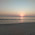@Venkatesh K
If the voltage applied would be equal to the rated value of voltage then there are chances of the rotor getting locked at the synchronous speed and hence we won't be able to determine the quadrature and direct axis reactance, which is the aim actually to perform slip test.

6.ok thank you @Aditya Dholakia

in the slip test we manitained at certain speed which is less than the synchronous speed.Then how can it is possible to maintain magnetic locking during the slip test.The name it self tell that there is a slip between rotor & stator

7.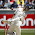slip test is conducted on which type of rotor and why??

1.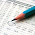Salient pole type.In cylindrical type there Xs does not vary.

8.MR.SWAMI NAVEEN

It is conducted on salient pole rotor to find out the Xd (i.e direct axis reactance) & Xq (i.e quadrature axis reactance)

9.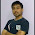Why reduced voltage?
As the field wdg is open initially there will be no back emf.Hence if applied rated armature voltage heavy current flows as there is no opposition and insulation of wdg will damage

1.This comment has been removed by the author.

2.3.But field winding is open, how can heavy current flow in it

10.Why reduced voltage?
As the field wdg is open initially there will be no back emf.Hence if applied rated armature voltage heavy current flows as there is no opposition and insulation of wdg will damage

11.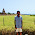Why we open field winding ??

12.Why we open field winding ??

1.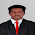You should connect voltmeter also

13.14.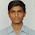@Vikas7was
(replay on gmail or on yahoo "vikas7was@gmail.com"or vikas7was@yahoo.in" or https://fb.com/vikas7was

Can we apply more then synchronous speed because on that case there will be slip between rotating magnetic field and rotar

15.16.why should we determine reactance in slip test